﻿ Spring Constant - showmethephysics.com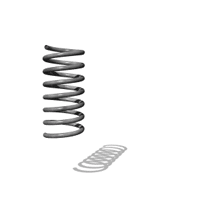HomeTry this at home!

What crushed this can?

S.M.U. Physics

The can implodes because the atmospheric pressure is greater than the pressure inside the can.

When the can was submerged in the water it caused the water vapor inside the can to rapidly cool.  When a gas is cooled, its pressure goes down. This drop in pressure causes the pressure inside the can to be lower than the atmospheric pressure.

Note: Use less than 1 cm of water in this experiment (about 1/2 inch)

showmethephysics.com

Elastic P.E. - work stored in a deformed spring

a) General Equation

PEs = Fx

F = Average Force needed to deform spring

x = distance deformed (stretch or compress)Ideal Spring

showmethephysics.com

b)  Hooke's Law - force needed to deform an ideal spring, a given amount is directly proportional to its displacement (deformation)Graphic from Learning-Connections ClipartRobert Hooke

'Newton's bitter rival'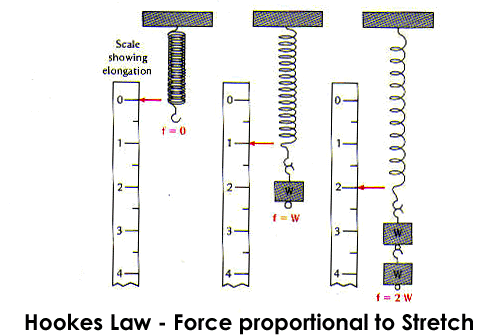Ex) Ideal Spring

 F (Force to Deform) (N) X (distance deformed) (m) 2.0 .30 4.0 .60 6.0 .90 8.0 1.2Hooke's Law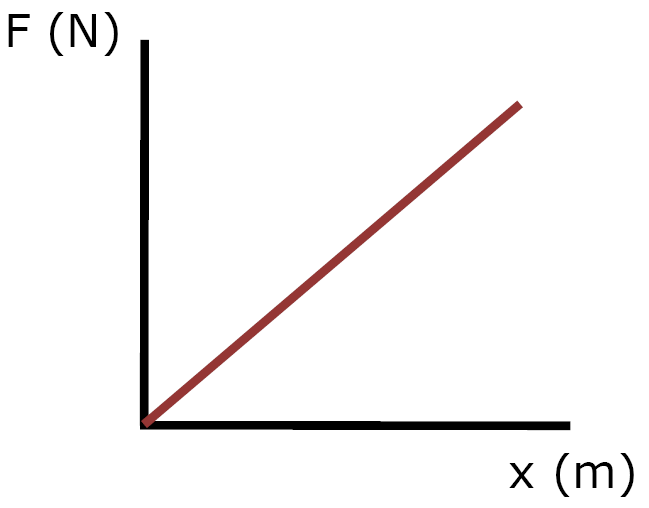slope = F/x

x = stretch

c) Spring Constant (k), Ideal Spring

- quantifies stiffness of a spring

 K = F (N) X (m)

What is the spring constant of the spring that produced the data? above?

 F (Force to  Deform) (N) X (distance deformed) (m) 2.0 .30 4.0 .60 6.0 .90 8.0 1.2

 k = 2.0 N .30 m

k = 6.7 N/m

Which is the stiffer spring?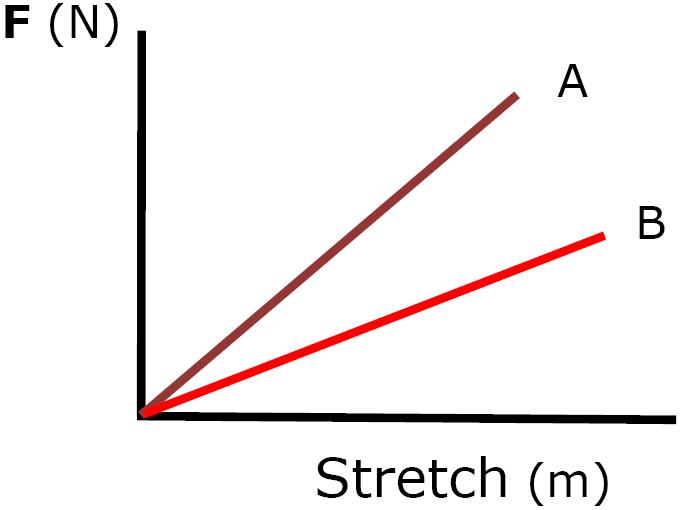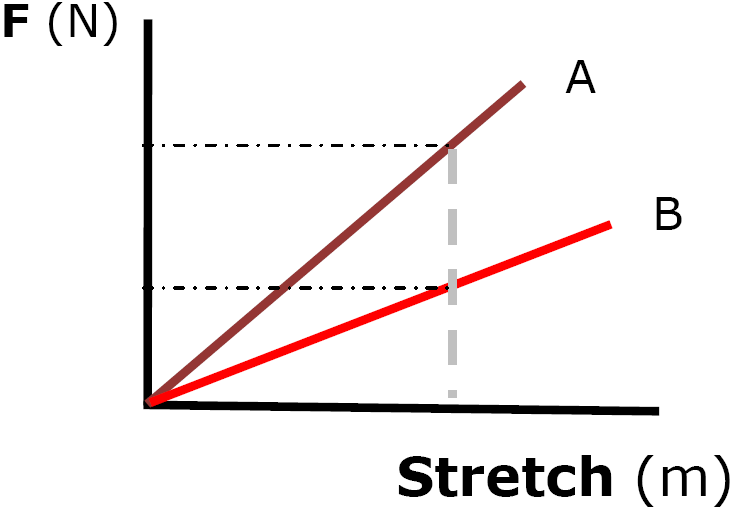Spring A is the stiffer spring.

More force is required
for the same stretchEx) Plots and KPick any point on the line

 K = F (N) X (m)

 K = 20. N .40 m

K = 50. N/m

d) Elastic P.E. = area of triangle under F vs x graph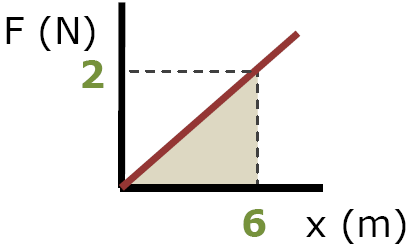PEs = (½)basexheight

PEs = 6 JoulesPE and kTry this at home!